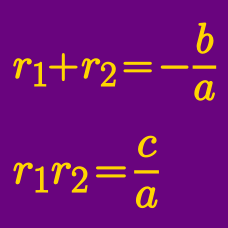Algebra

# Vieta's Formula - Higher Degrees

$\alpha$, $\beta$, and $\gamma$ are the roots of the cubic equation $x^3-x^2+2x-13=0$. If $\frac{1}{\alpha}+\frac{1}{\beta}+\frac{1}{\gamma}=\frac{a}{b}$, where $a$ and $b$ are coprime positive integers, what is the value of $a+b$?

Given that 2 roots of $f(x) = x^3 + ax + b$ are 2 and 7, what is $a+b$?

What is the product of all roots to the equation

\begin{aligned} & (x-1)(x-2)(x-3) + (x-2)(x-3)(x-4) \\ + & (x-3)(x-4)(x-5) + (x-4)(x-5)(x-6) \\ + & (x-5)(x-6)(x-7) + (x-6)(x-7)(x-8) =0 ? \end{aligned}

Details and assumptions

Clarification: Make sure you scroll to the right (if need be) to see the full equation. This problem ends with a "?".

If the quartic $x^4 + 3x^3 + 11 x^2 + 15 x + A$ has roots $k, l, m$ and $n$ such that $kl = mn$, determine $A$.

If the cubic equation $x^3-3x^2+5=0$ has three roots $\alpha$, $\beta$ and $\gamma$, what is the value of $(\alpha+\beta)(\beta+\gamma)(\gamma+\alpha)?$

×